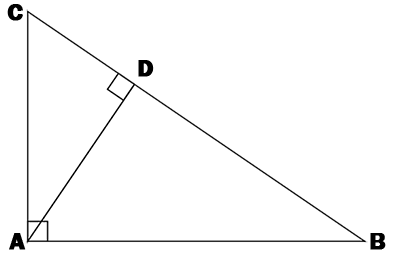### A right triangle ABC has the hypotenuse BC = 52 cm and the height AD is perpendicular to BC. If BD = 36 cm, calculate the length of the height AD.Fill in herecm

### Remember !

Altitude definition theorem. In a right triangle, the length of the height joining from the tip of the right angle to the projection on the hypotenuse is equal to the geometric mean between the lengths of the projections of the cathetus on the hypotenuse.Abbreviations: A - area, AB - cathetus, AC - cathetus, BC - hypotenuse and AD - height.

### Exemple !

A right triangle ABC has the hypotenuse BC = 13 cm and the height AD is perpendicular to BC. If BD = 4 cm, calculate the length of the height AD.

BC = BD + DC

DC = BC - BD

DC = 13 - 4 = 9 cm

We use the Altitude Theorem